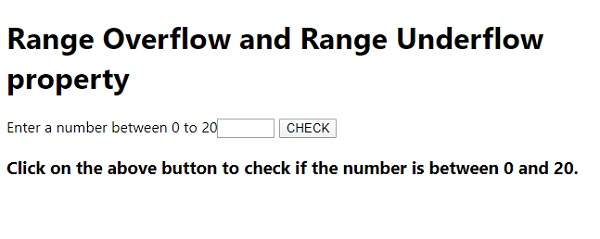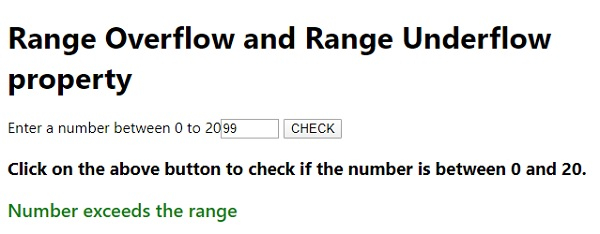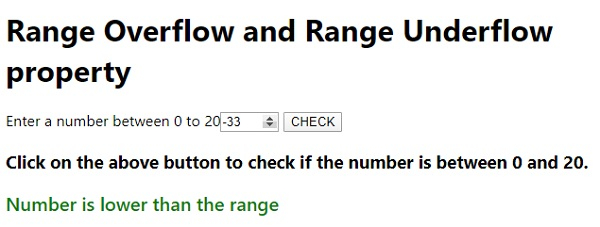# Range Overflow and Range Underflow properties in JavaScript.

Range Underflow − If the element value is less than that of the specified in min attribute, it sets to true.

Range Overflow − If the element value is more than that of the specified in max attribute, it sets to true.

Following is the code for Range overflow and range underflow property in JavaScript −

## Example

Live Demo

<!DOCTYPE html>
<html lang="en">
<meta charset="UTF-8" />
<meta name="viewport" content="width=device-width, initial-scale=1.0" />
<title>Document</title>
<style>
body {
font-family: "Segoe UI", Tahoma, Geneva, Verdana, sans-serif;
}
.result {
font-size: 20px;
font-weight: 500;
}
</style>
<body>
<h1>Range Overflow and Range Underflow property</h1>
Enter a number between 0 to 20<input type="number" class="num" min="0" max="20"
step="1"></div>
<button class="Btn">CHECK</button>
<h3>
Click on the above button to check if the number is between 0 and 20.
</h3>
<div style="color: green;" class="result"></div>
<script>
let resEle = document.querySelector(".result");
let numEle = document.querySelector('.num');
if(numEle.validity.rangeOverflow){
resEle.innerHTML = 'Number exceeds the range';
}
else if(numEle.validity.rangeUnderflow){
resEle.innerHTML = 'Number is lower than the range ';
}
else{
resEle.innerHTML = 'Number lies in the range';
}
});
</script>
</body>
</html>

## Output

The above code will produce the following output −On entering a number above 20 −On entering a number below 0 −